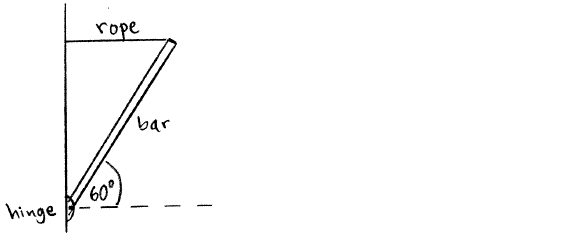# Problem: One end of a uniform bar that is 6.0 m long is attached to a vertical wall by a frictionless hinge. The bar is held at an angle of 60° above the horizontal by a horizontal rope that is attached to the other end of the bar, as shown in the sketch. If the tension in the rope is 120 N, what is the mass m of the bar?

91% (53 ratings)
###### Problem Details

One end of a uniform bar that is 6.0 m long is attached to a vertical wall by a frictionless hinge. The bar is held at an angle of 60° above the horizontal by a horizontal rope that is attached to the other end of the bar, as shown in the sketch. If the tension in the rope is 120 N, what is the mass m of the bar?